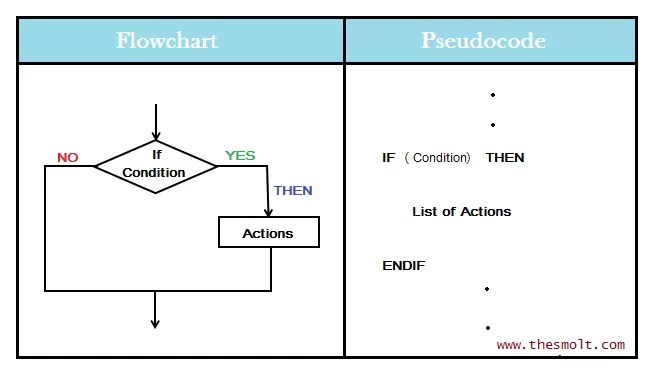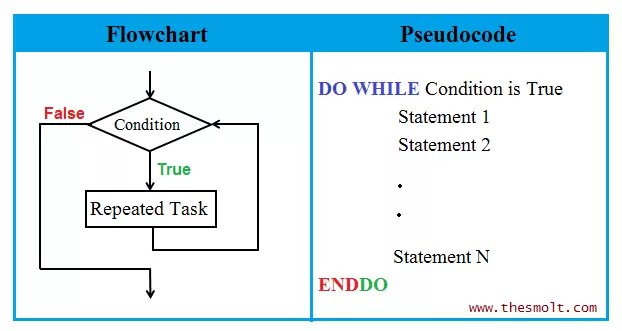# Concept of Drawing Flowchart

## Flowchart Structure & Concept of Flowchart

(Hello My Dear student today we’ll learn Concept of Drawing Flowchart in this Article)

flowchart can be simple where only few boxes and lines depict the entire logic. That can be complex to where the logic deflection is really a nightmare. However no matter how simple or Complex the solution is there are mainly three key concepts which are used in drawing a flowchart.

1. Sequence  (where information can flow in a straight line).
2. Selection (where the decisions are made according to some predefined condition).
3. Reputation (where the logic can repeat in a loop that is where a sequence of steps                          in repeated until the desired output is obtained).

###### Sequence:-

As the name implies in a sequence structure the instruction to be computed follow one another in a logical progression. On a flowchart the symbol representing each instruction has only a single entering path and a single existing path. Typical sequence operation consist of process and input output steps.Above example the first symbol is a terminal symbol with text is ‘Start’. This symbol indicates the beginning of the flowchart. This second symbol is an input output symbol text as ‘A=10, B=20′ , which indicates the initialization of two numbers (A and B) to  10 and 20, respectively. Third symbol is a processing symbol labelled as ‘C= A+B‘. In this steps the processing that is addition is educated and the sum is stored in a memory variable, which is given the name C. The fourth symbol is again in input / output symbol which is labelled as ‘Print C’. This step will print the output (value of C) on an output device such as monitor. The Last symbol text as ‘Stop’, indicates the conclusion of the problem solving logic.

###### Reflection:-

Reflection structure allows the program to make a choice between two alternate path depending upon the condition, that is, whether it is true or false. The first statement of a selection structure is a conditional statement that obviously involves a decision symbol when they represented on a flowchart. Once the sequence of steps in the selected path has been carried out, the paths are rejoined and then the next instruction, after the connector, is carried out. Thus, taken as a whole, the selection instructor has only a single entry and a single entry and a single exit. It is therefore, could be represented on a flowchart as a single operational block in the sequence structure.

###### Repetition:-It causes in interruption in the normal processing of sequence, and direct the system to look back to a previous statement in the program, repeating the same sequence over and again, usually with new data. When a sequence of a statement is repeated against a condition it is said to be in a loop. Using looping, the programmer avoids repeating the same set of instruction. The looping process can be either one time or multiple times until the desired output is obtained within a single program.Dear Student,